### Introduction to Earth Data Analysis

1. Get Started
1. Learn Git/Github for team collaboration
2. Install Python via Anaconda
3. Install Python IDE (Jupyter Notebook, Visual Studio Code)
4. Python variables and data types
5. Install and use Python packages
6. Get familiar with Numpy for data manipulations
7. Get familiar with Matplotlib for plotting arrays
8. Get familiar with Pandas to analyze tabular data
2. Get familiar with text file formats
1. Use basic Markdown syntax to format text in Jupyter Notebook files
2. Get familiar with text file formats - CSV, .txt, YAML
3. Read/Write data from text files using Python
4. Read/Write data from text files using Pandas
5. Deal with missing data in Pandas
3. Spatial Data Analysis
1. Read multi-layered raster data (.tif / .hdf / .nc) in Python
2. Read vector data (shapefiles) using geopandas
3. GIS in Python
4. Time-series Analysis using Pandas
2. Work with `Datetime` formats
3. Resample time series
4. Filtering/smoothing time-series with Pandas
5. Plot time series
5. How to plot topographic high-resolution geospatial maps

## Recent posts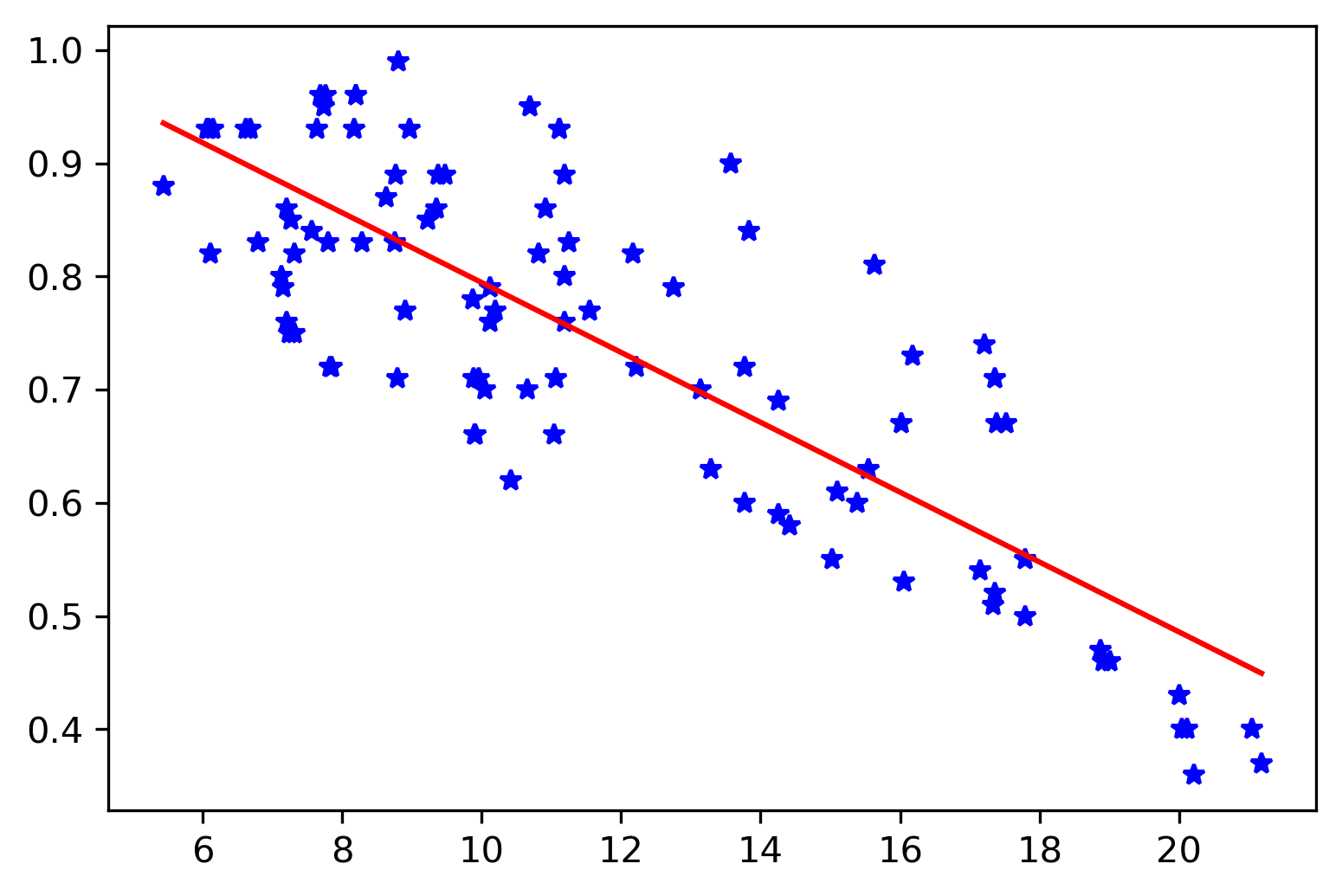## Maximum likelihood estimation for the regression parameters

We will learn the basics of the maximum likelihood method, and then apply it on a regression problem. We will also compare it with the least-squares estimati...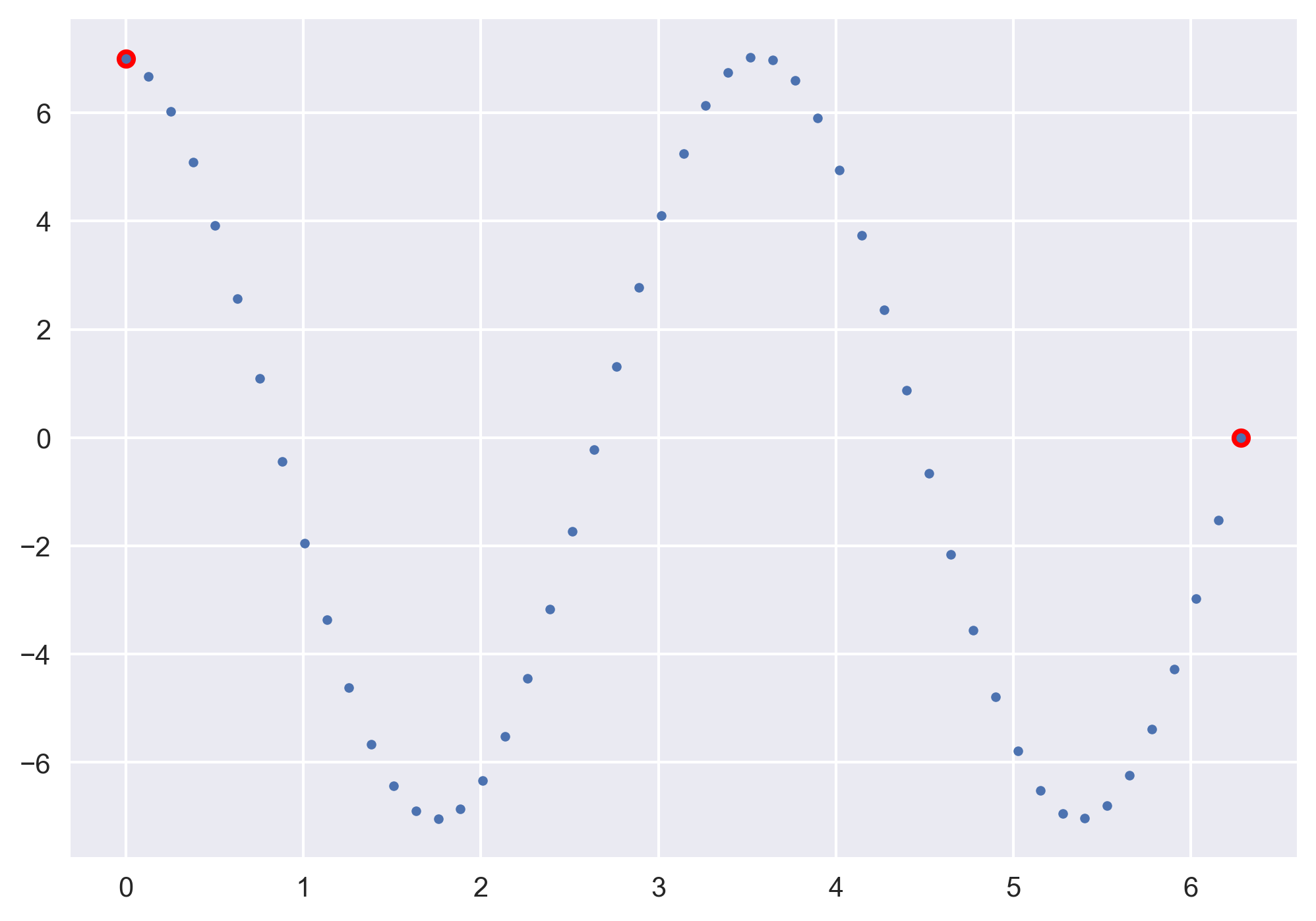## Solving boundary value problems using the shooting method

The boundary value problems require information at the present time and a future time. We will see how we can use shooting method to solve problems where we ...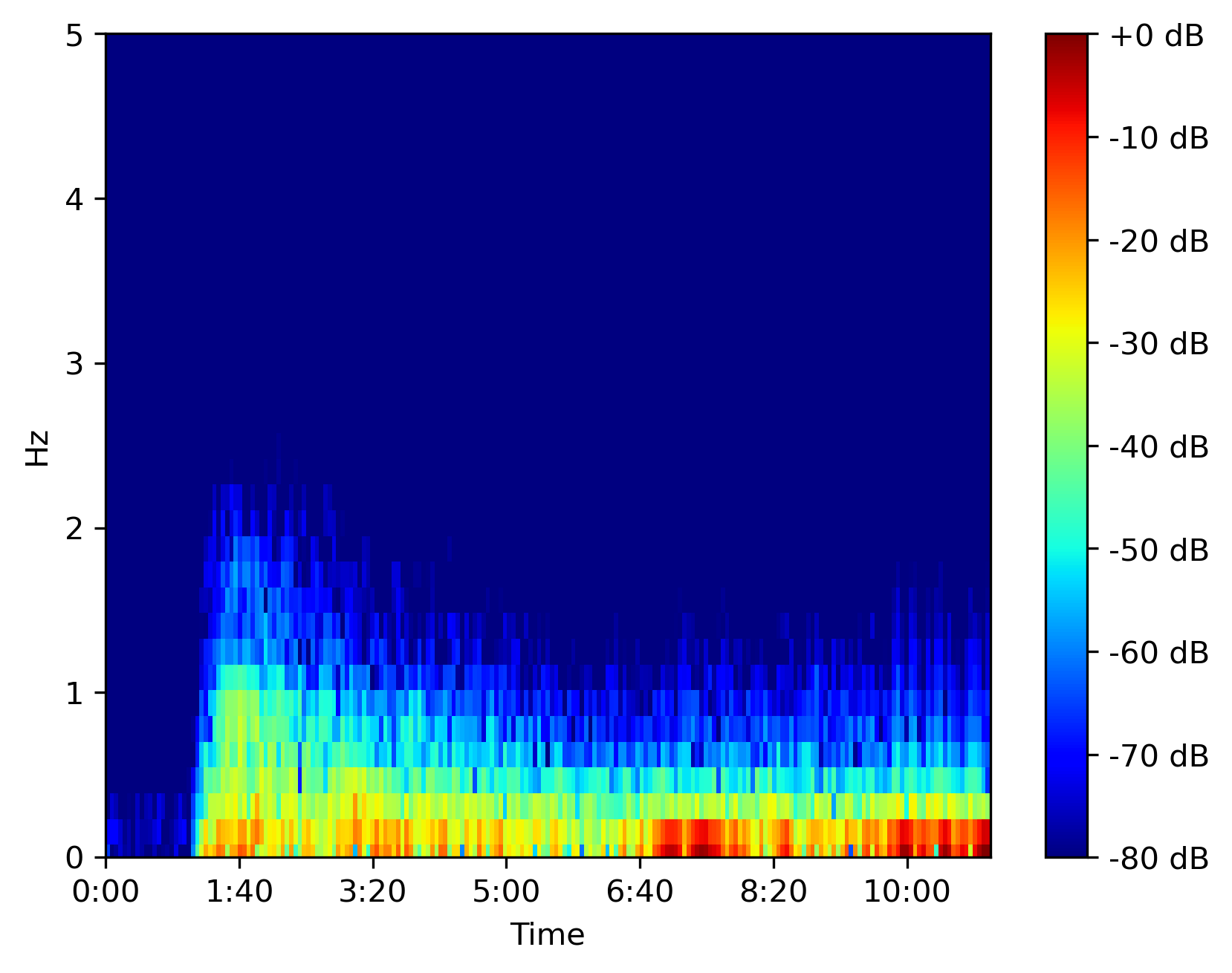## Efficiently compute spectrogram for large dataset in python

Librosa can efficiently compute the spectrogram for large time series data in seconds. We will use that to plot the spectrogram using matplotlib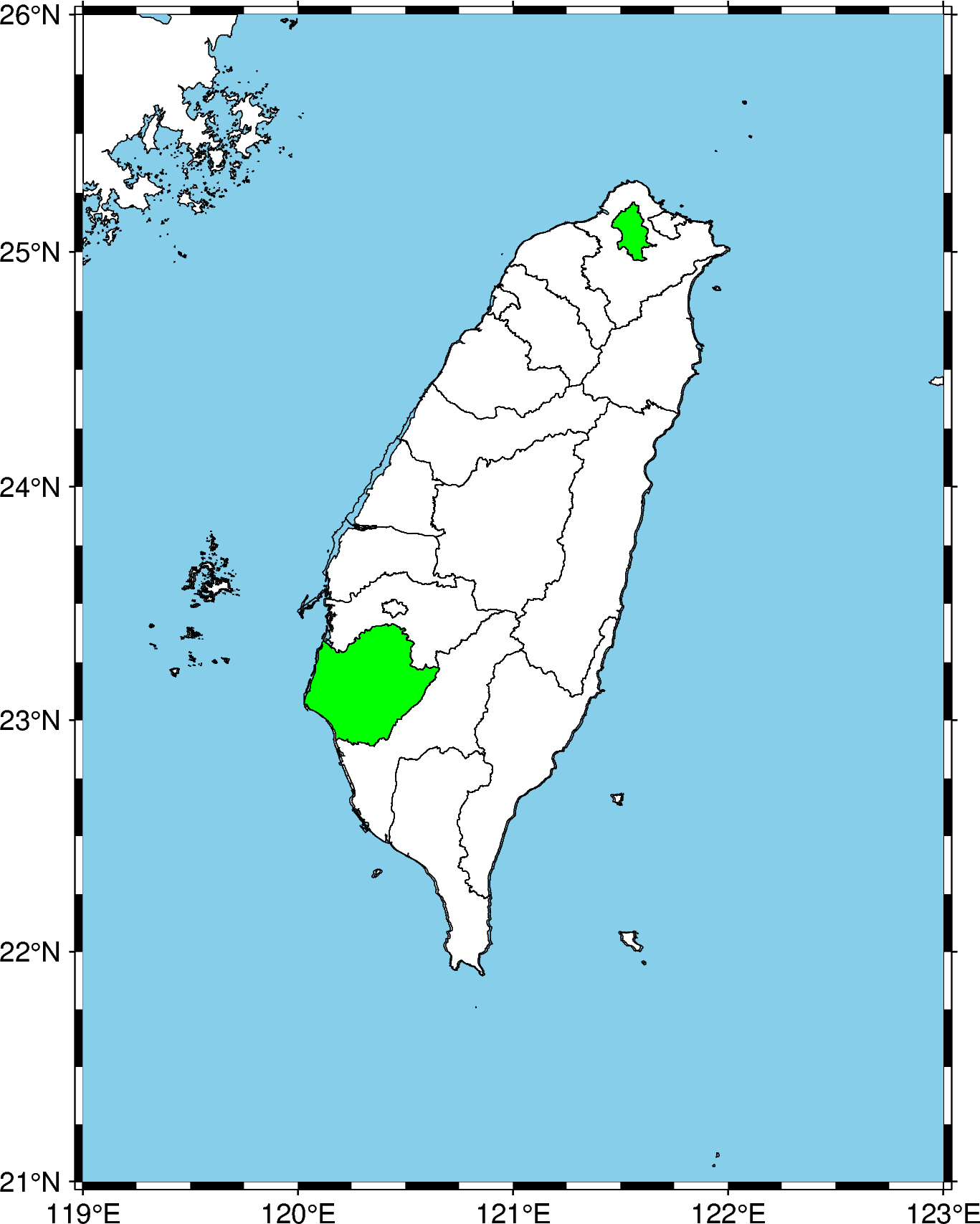## How to overlay shapefile data on pygmt maps

We learn how to plot selected shapefile data using geopandas on top of PyGMT maps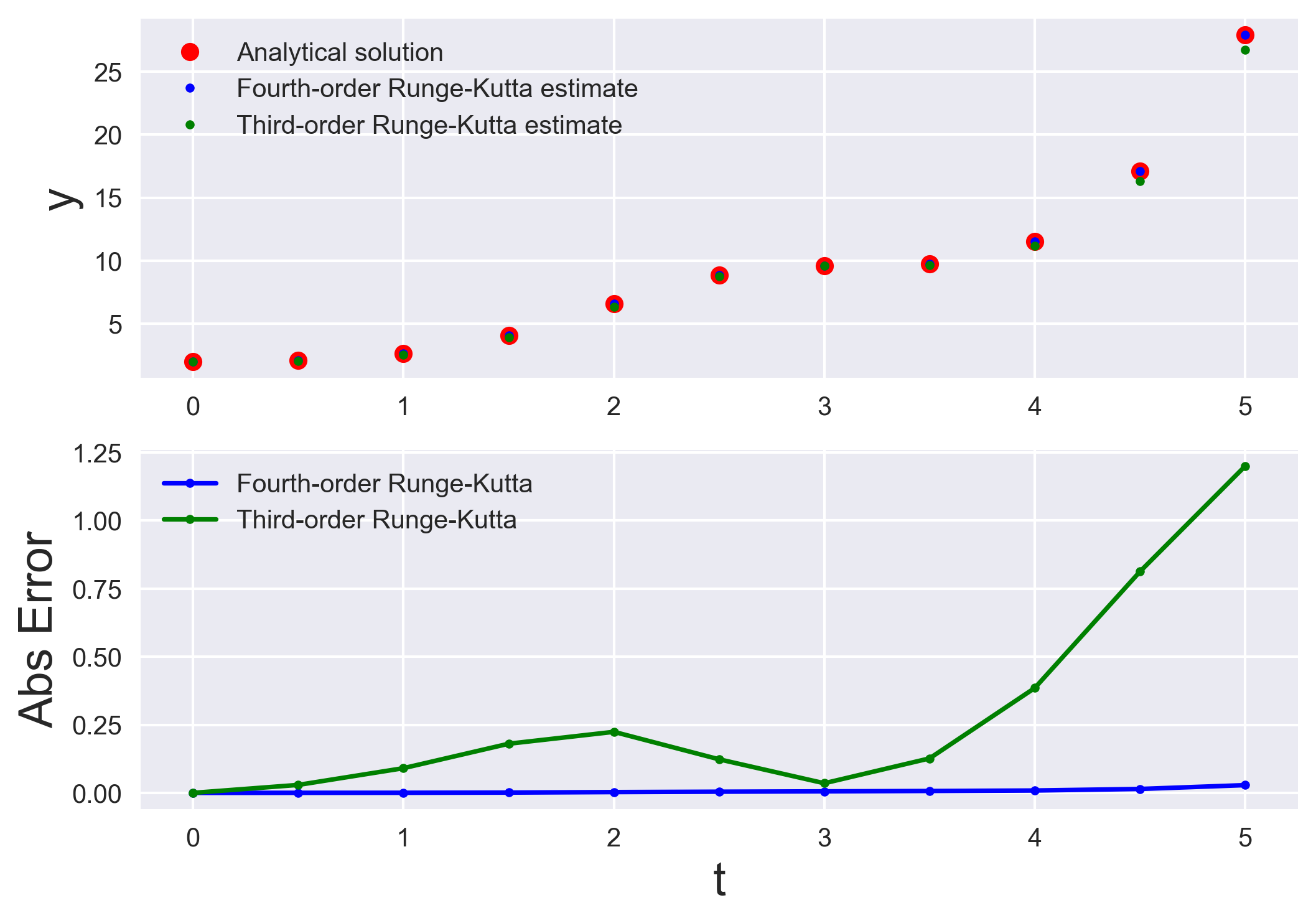## Numerically solving initial value problems using the runge-kutta method

Runge-Kutta methods are most popular method to solve ordinary differential equations (ODEs) with a better approximation than the Euler method. We compare the...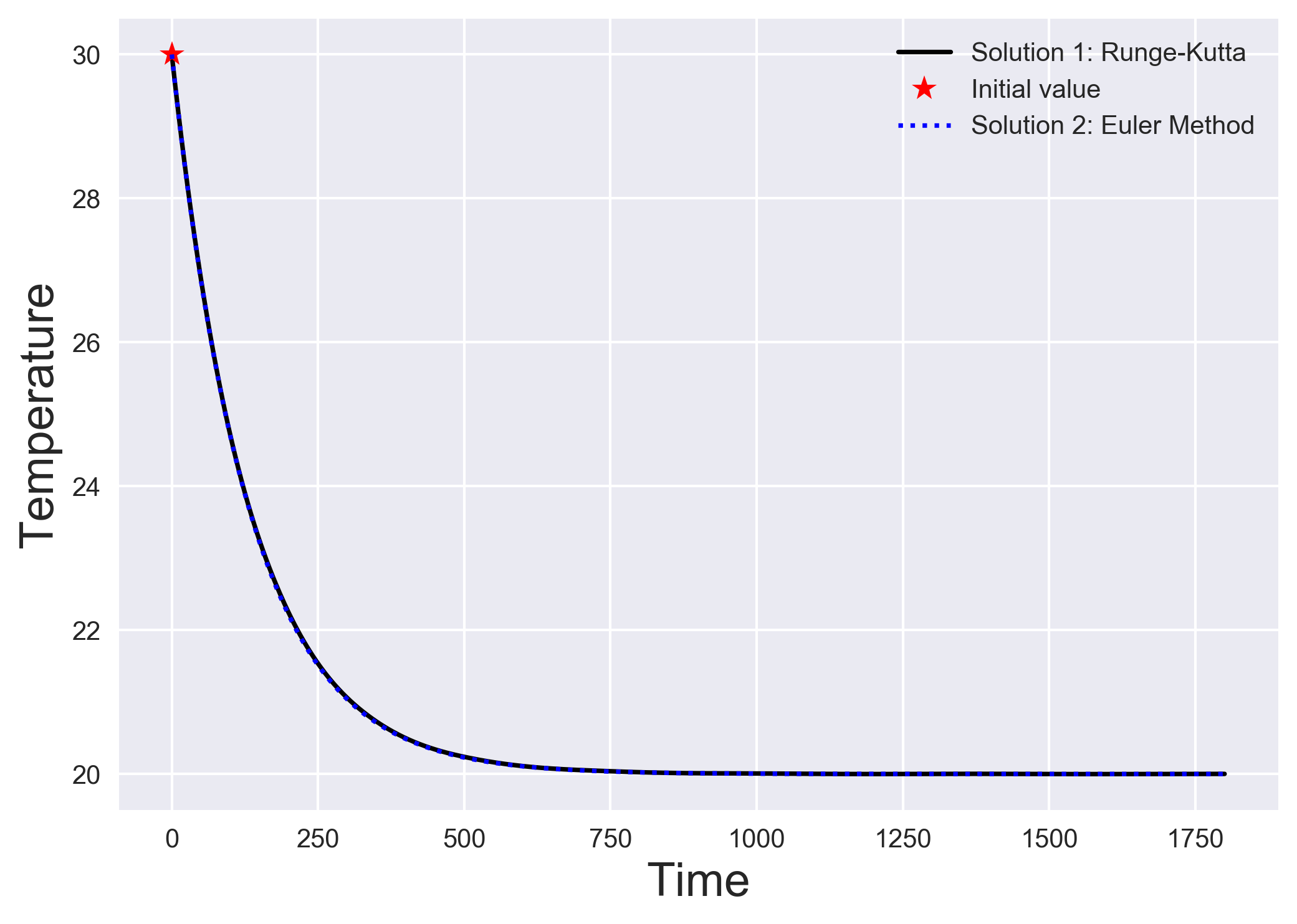## Numerically solving initial value problems using the euler method

The simplest algorithm to solve a system of differential equations is the Euler method. We understand the Euler method by looking into a simple heat transfer...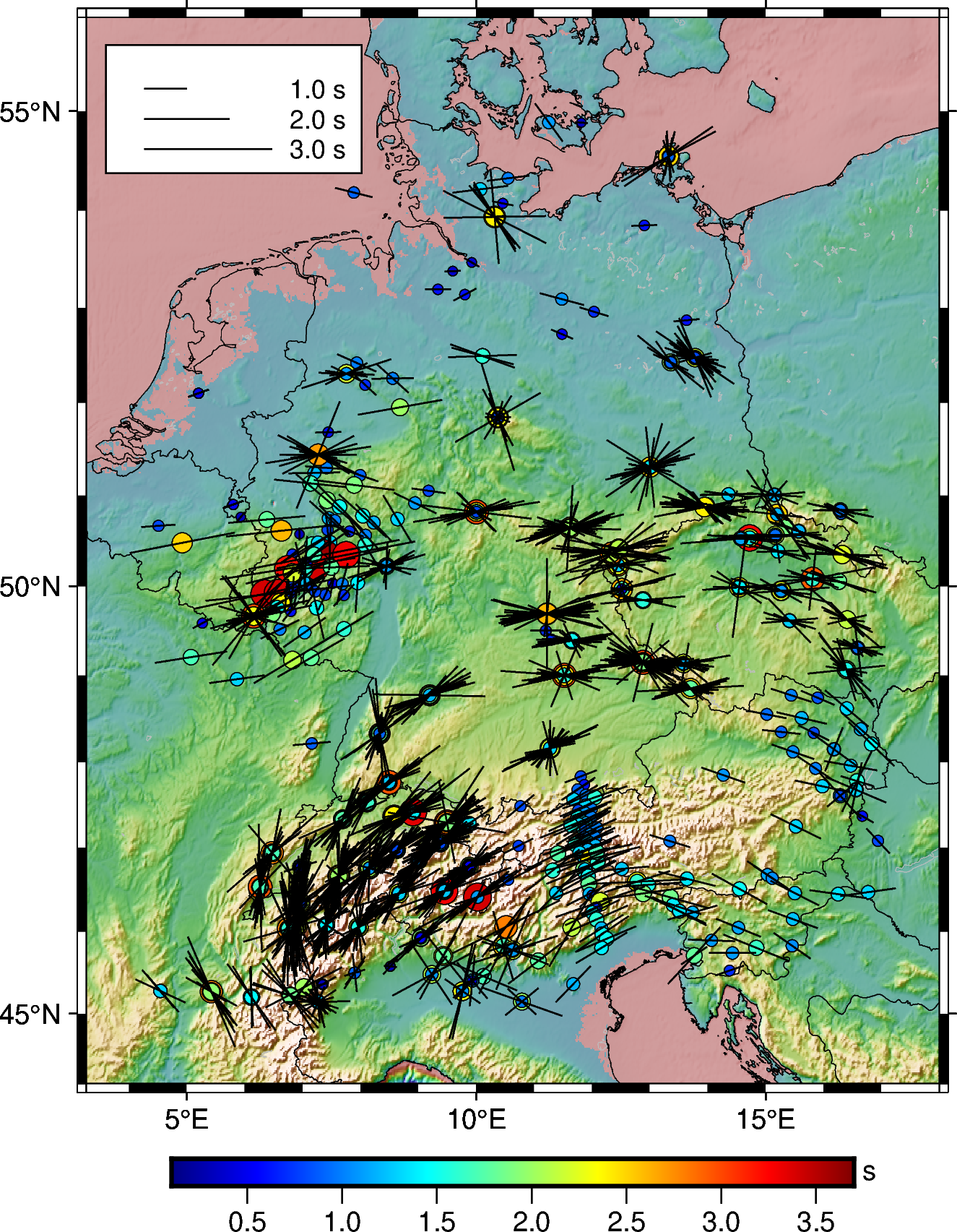## How to plot shear-wave splitting measurements using pygmt

How you can plot the shear-wave splitting measurements from splitting database using PyGMT.How can you link unsplash images directly to your blog without hosting it locally.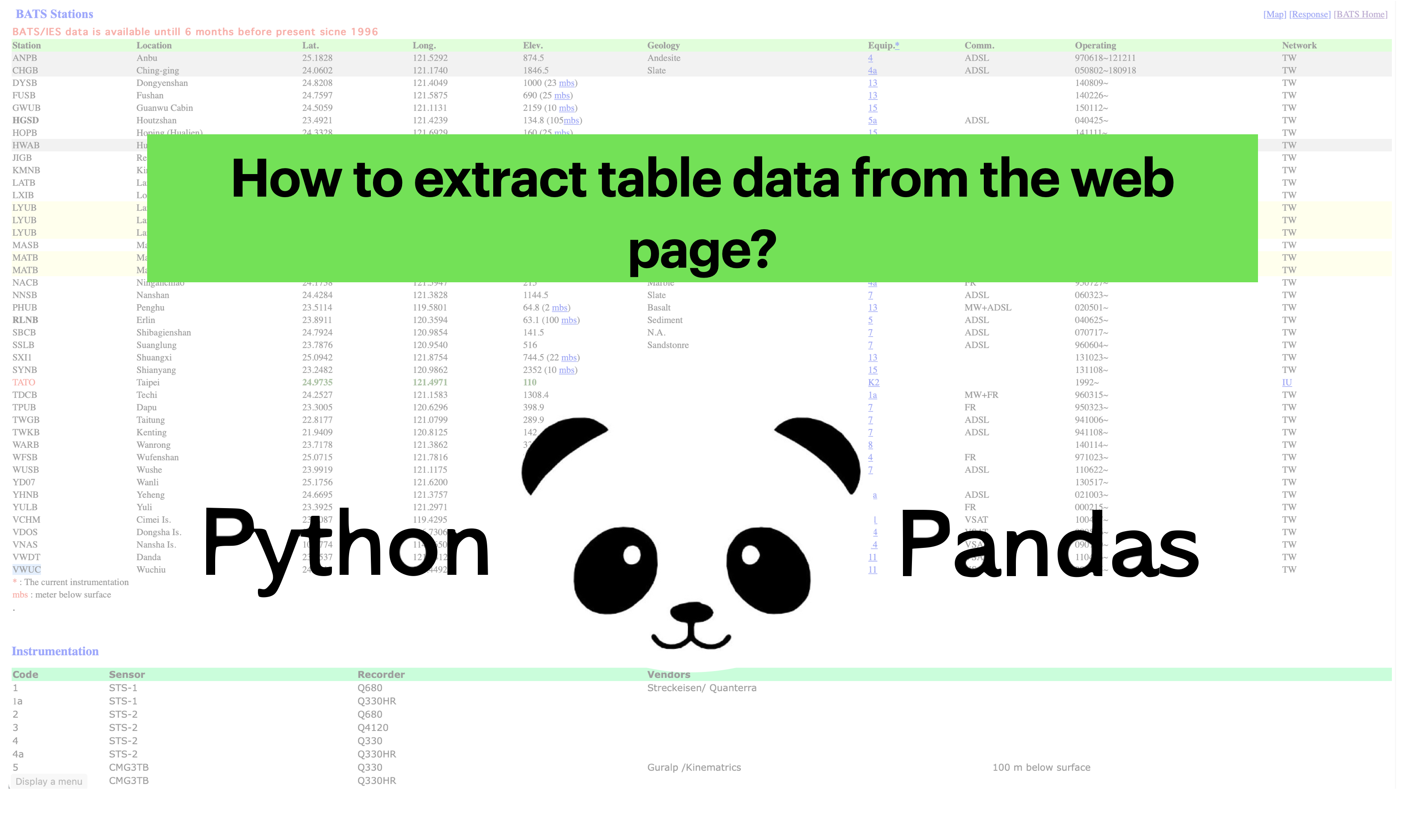## How to extract station data from the web page

Uses pandas to read the html page and extract the html data into pandas dataframe## How to plot the earthquakes data on a topographic map

Read the earthquake data from a csv file and overlay on a topographic map using PyGMT.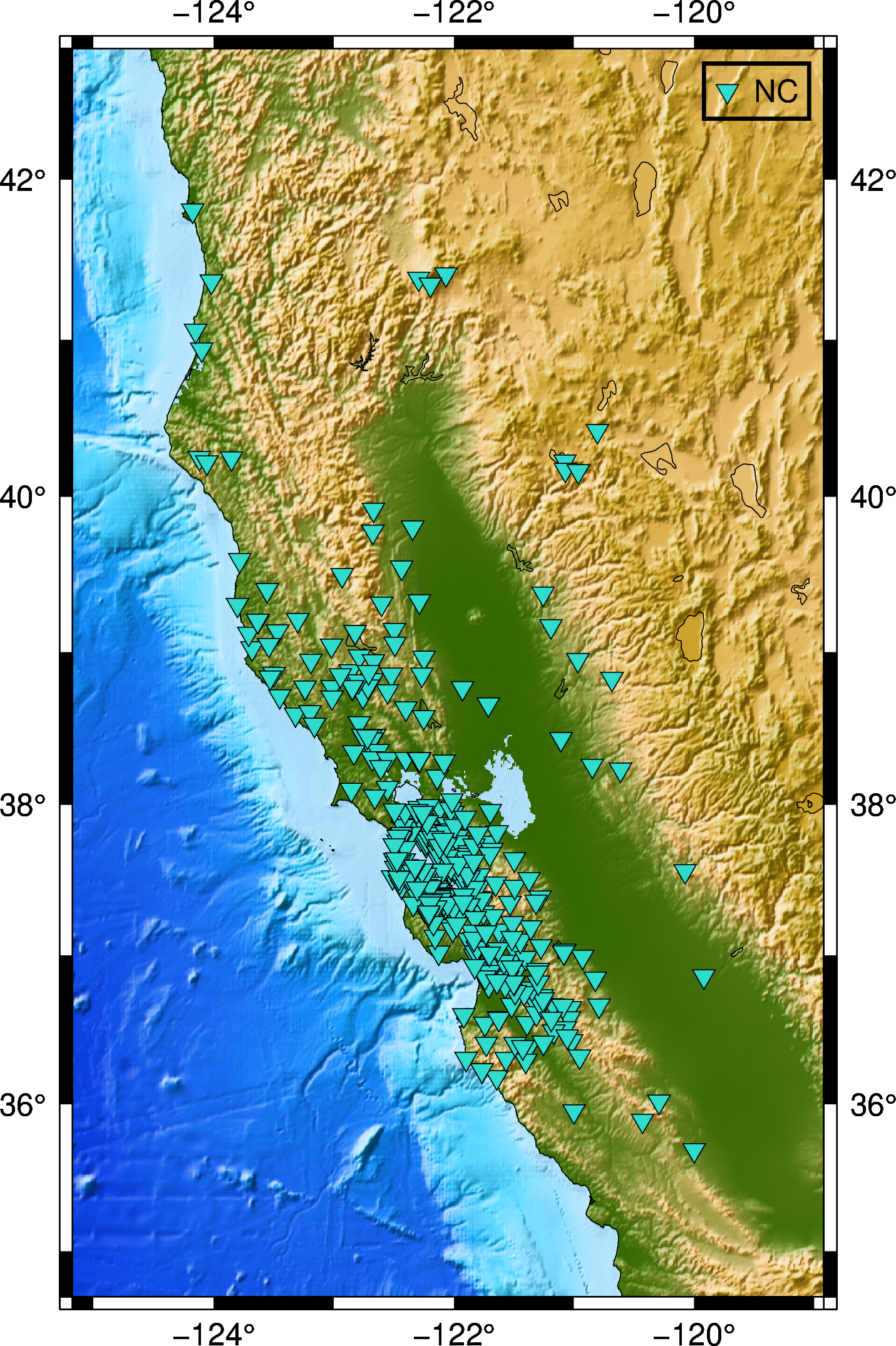## How to automatically enquire the availability of seismic data using obspy

In this post, we will see how can we retrive the available seismic waveforms information for a given network, station, channel and client in a given period o...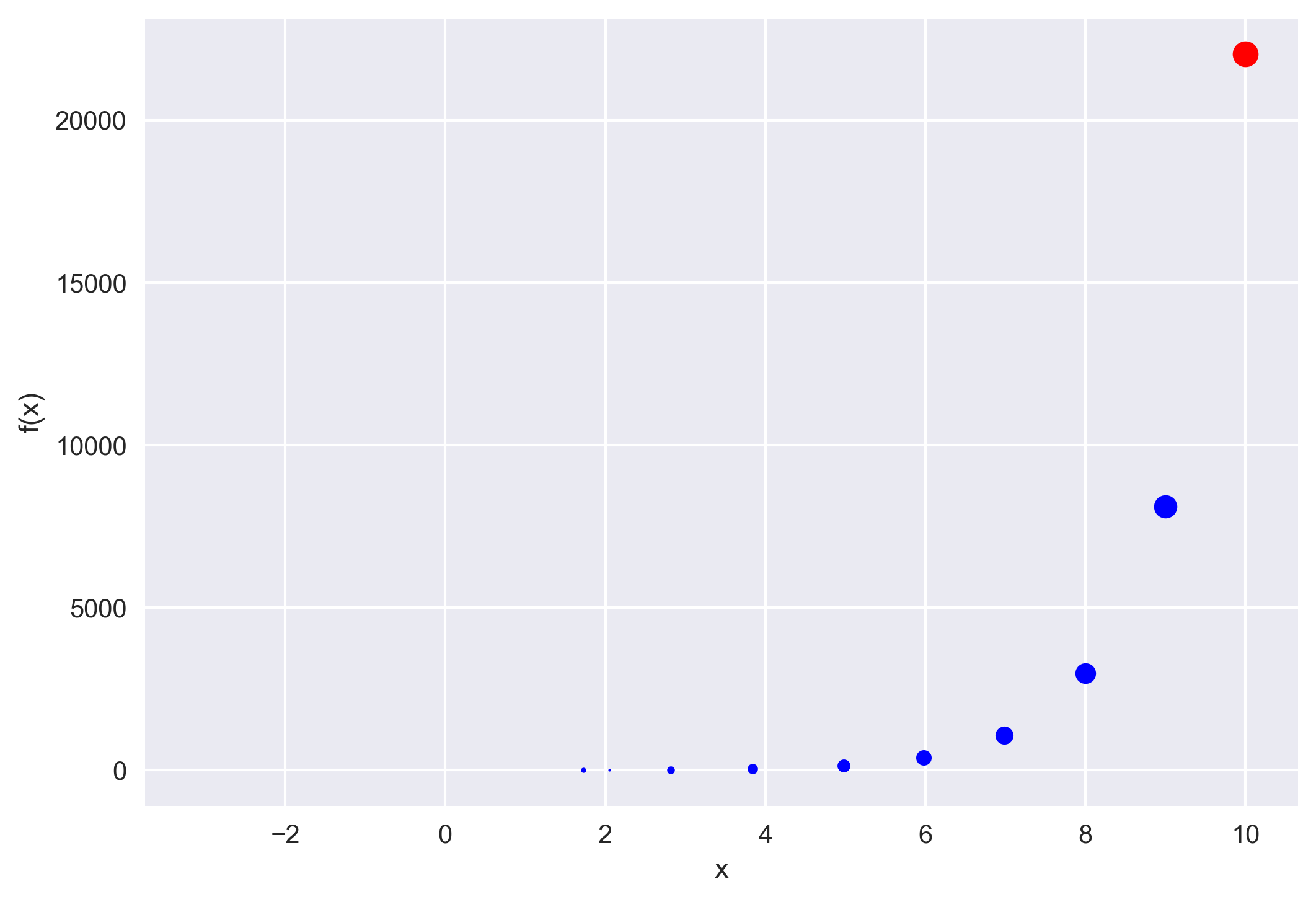## How to implement the iterative newton–raphson method to find roots of a function in python

The Newton–Raphson method (commonly known as Newton’s method) is developed for finding roots of a given function or polynomial iteratively. We show two examp...## How to install jekyll on apple m1 macbook

If you have recently bought a M1 mac and have been doing blogging using Jekyll, then you must have experienced that installing Jekyll on the M1 architecture ...## How to create a simple makefile in linux

We learn how to write a Makefile to automate the compilation of our source code. We will use one example from Fortran.## Maximum likelihood estimation for the regression parameters

We will learn the basics of the maximum likelihood method, and then apply it on a regression problem. We will also compare it with the least-squares estimati...## Solving boundary value problems using the shooting method

The boundary value problems require information at the present time and a future time. We will see how we can use shooting method to solve problems where we ...## Efficiently compute spectrogram for large dataset in python

Librosa can efficiently compute the spectrogram for large time series data in seconds. We will use that to plot the spectrogram using matplotlib## How to overlay shapefile data on pygmt maps

We learn how to plot selected shapefile data using geopandas on top of PyGMT maps## Numerically solving initial value problems using the runge-kutta method

Runge-Kutta methods are most popular method to solve ordinary differential equations (ODEs) with a better approximation than the Euler method. We compare the...## Numerically solving initial value problems using the euler method

The simplest algorithm to solve a system of differential equations is the Euler method. We understand the Euler method by looking into a simple heat transfer...## How to plot shear-wave splitting measurements using pygmt

How you can plot the shear-wave splitting measurements from splitting database using PyGMT.How can you link unsplash images directly to your blog without hosting it locally.## How to extract station data from the web page

Uses pandas to read the html page and extract the html data into pandas dataframe## How to plot the earthquakes data on a topographic map

Read the earthquake data from a csv file and overlay on a topographic map using PyGMT.## How to automatically enquire the availability of seismic data using obspy